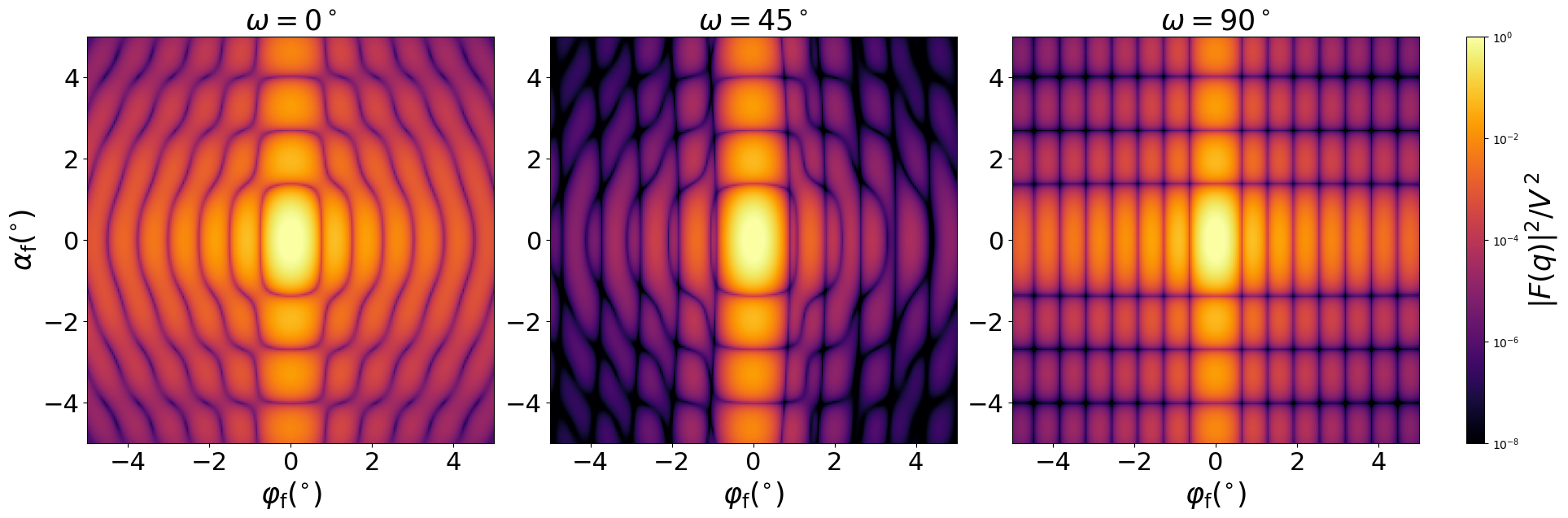### HorizontalCylinder

A horizontal truncated circular cylinder.

#### Constructor

Full form python HorizontalCylinder(R, H, b, t)

and short form
python
HorizontalCylinder(R, H) == HorizontalCylinder(R, H, -R, R)


Parameters:

• H, height
• b, bottom boundary, relative to the center
• t, top boundary, relative to the center

Constraint:

$-R \le b \le t \le R$

#### Usage

As for any other Form factor.

#### Implementation

Class HorizontalCylinder inherits from the interface class IFormFactor .

Form factor is computed as

$$F(\mathbf{q})=2 L \space \text{sinc}\Big(q_x\dfrac{L}{2}\Big) \int_{b}^{t} \text{d}z \space \sqrt{R^2-z^2} \space \text{sinc}(q_y\sqrt{R^2-z^2}) \exp[iq_z(z-b)] ,$$

Volume has been validated against $$V=L \Big( t\sqrt{R^2-t^2}- b\sqrt{R^2-b^2} + R^2 \Big[\text{asin}\Big(\frac{t}{R}\Big) - \text{asin}\Big(\frac{b}{R}\Big) \Big] \Big).$$

More general:

• Cylinder, if vertical and non-truncated.

#### Example

Scattering by uncorrelated, oriented horizontal truncated cylinders for horizontal incidence. Rotation around $z$ axis:Generated by Examples/ff/HorizontalCylinder.py .

#### History

Introduced in BornAgain 1.20.